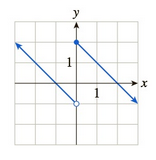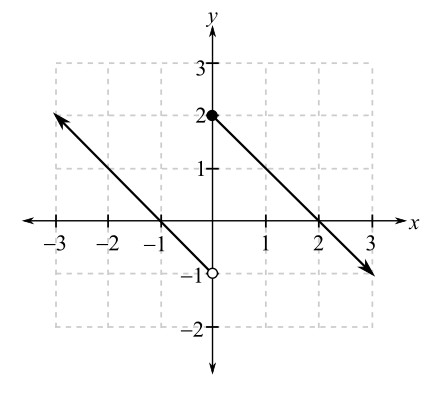Chapter 10.1, Problem 39EFinite Mathematics and Applied Cal...

7th Edition
Stefan Waner + 1 other
ISBN: 9781337274203

Solutions

Chapter
SectionFinite Mathematics and Applied Cal...

7th Edition
Stefan Waner + 1 other
ISBN: 9781337274203
Textbook Problem

In Exercises 35–48 the graph of f is given. Use the graph to compute the quantities asked for. [HINT: See Examples 4–5.]a. lim x → 2 f ( x ) b. lim x → 0 + f ( x ) c. lim x → 0 − f ( x ) d. lim x → 0 f ( x ) e. f ( 0 ) f. lim x → − ∞ f ( x )(a)

To determine

The value of limx2f(x) using the graph which is as follows:Explanation

Given information:

The graph is as follows:

From the graph, evaluate the limit for x tends to 2 for the function f(x).

For this, take a point on the graph to the left of x=2 and move along the curve, so that the x-coordinate approaches 2. Then, it is noticed from the graph that the y-coordinate approaches 0.

Similarly, take a point on the graph to the right of x=2 and when move along the curve to the left so that the x-coordinate approaches 2

(b)

To determine

The value of limx0+f(x) using the graph which is as follows:(c)

To determine

The value of limx0f(x) using the graph which is as follows:(d)

To determine

The value of limx0f(x) using the graph which is as follows:(e)

To determine

The value of f(0) using the graph which is as follows:(f)

To determine

The value of limxf(x) using the graph which is as follows:Still sussing out bartleby?

Check out a sample textbook solution.

See a sample solution

The Solution to Your Study Problems

Bartleby provides explanations to thousands of textbook problems written by our experts, many with advanced degrees!

Get Started

Evaluate the limit. limx(/2)(tanx)cosx

Single Variable Calculus: Early Transcendentals, Volume I

Evaluate the integrals in Problems 23-26, using the formulas in Table 13.2. 25.

Mathematical Applications for the Management, Life, and Social Sciences

True or False: is a convergent series.

Study Guide for Stewart's Single Variable Calculus: Early Transcendentals, 8th